## Recommended Posts

For this challenge, I decided to go with something most everyone is familiar with, the parabola.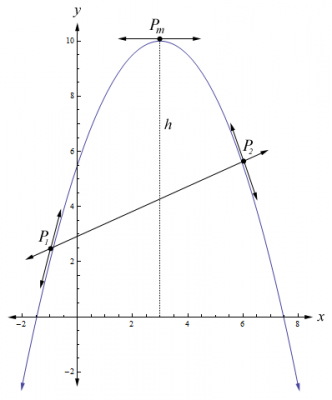Given that $y=a x^2 + b x + c$, the goal of this challenge is to find the parabolas that reach a height of $h$, and go through the points, $P_1=\{x_1,\ y_1\}$ and $P_2=\{x_2,\ y_2\}$. All of the variables have been specified, and although this challenge may seem easy, I assure you that it is quite difficult. Reputation awaits the one who can provide the solution for this challenge first.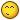Edited by Daedalus

##### Share on other sites

For this challenge, I decided to go with something most everyone is familiar with, the parabola.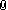Parabola.png

Given that $y=a x^2 + b x + c$, the goal of this challenge is to find the parabolas that reach a height of $h$, and go through the points, $P_1=\{x_1,\ y_1\}$ and $P_2=\{x_2,\ y_2\}$. All of the variables have been specified, and although this challenge may seem easy, I assure you that it is quite difficult. Reputation awaits the one who can provide the solution for this challenge first.Wait, so we have to remake that parabola?

##### Share on other sites

Wait, so we have to remake that parabola?

No, the challenge is to find both parabolas that pass through the points, $P_1$ and $P_2$, and reach a maximum height of $h$

Edited by Daedalus

##### Share on other sites

Despite the difficulty mentioned, I highly encourage anyone interested to try and solve this challenge. After all, it is based on parabolas and uses the quadratic formula. Thus, you only need algebra to solve it.Here is a graph that I have produced illustrating the problem, which should clarify the goals for the challenge.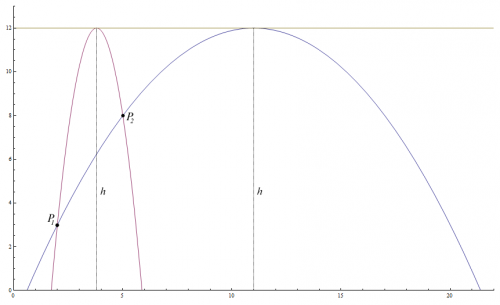As you can see, both parabolas pass through the points, $P_1$ and $P_2$, and reach a maximum height of $h$.

Edited by Daedalus

##### Share on other sites

I think I have a solution, but I'm not sure if I understand the scenario correctly...

So we have two distinct quadratics with equal height but nothing more specific, and two distinct points but nothing more specific, i.e. all expressions and functions are generalized?

##### Share on other sites

I think I have a solution, but I'm not sure if I understand the scenario correctly...

So we have two distinct quadratics with equal height but nothing more specific, and two distinct points but nothing more specific, i.e. all expressions and functions are generalized?

Yes, you will end up with two parabolas where $a$, $b$, and $c$ will be in terms of $\{x_1, y_1\}$, $\{x_2, y_2\}$, and $h$. However, both parabolas are actually a result of one equation, which is kind of obvious since both parabolas must pass through both points and reach a maximum height of $h$.

Edited by Daedalus

##### Share on other sites

I'd like to see solutions, please. My try was a total failure.I tried getting an equation of a parabola (y - h = A(x - a)^2) where A was in terms of the two points...

##### Share on other sites

I'd like to see solutions, please. My try was a total failure.I tried getting an equation of a parabola (y - h = A(x - a)^2) where A was in terms of the two points...

I always provide solutions whenever two weeks to a month have passed and the problem remains open, or when someone solves the challenge. However, I do provide hints to help you along the way. The first thing you should do is as follows:

Write the parabolas using the given points:

$y_1=a x_1^2+b x_1+c$

$y_2=a x_2^2+b x_2+c$

Next, you need to find a way to set them equal to each other so you can solve for $a$, $b$, and $c$.

Edited by Daedalus

##### Share on other sites

This is much trickier than I thought it would be.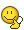I attempted a start by having the two quadratics in vertex form...

$f(x)=p(x-m)^2+h$

$g(x)=q(x-n)^2+h$

...with the $h$ parameter to secure the "equal heights" part, and also $p,q<0$ to ensure that it reflects the functions' maxima.

I tried to reconcile my start with your equation using corresponding terms, but the resulting system is very messy. Then, I tried your method purely, but I failed to solve for $a, b, c$ without running into an inconsistency or tautology. Blargh!

##### Share on other sites

This is much trickier than I thought it would be.I attempted a start by having the two quadratics in vertex form...

$f(x)=p(x-m)^2+h$

$g(x)=q(x-n)^2+h$

...with the $h$ parameter to secure the "equal heights" part, and also $p,q<0$ to ensure that it reflects the functions' maxima.

I tried to reconcile my start with your equation using corresponding terms, but the resulting system is very messy. Then, I tried your method purely, but I failed to solve for $a, b, c$ without running into an inconsistency or tautology. Blargh!

It is a very difficult problem to solve. I'll give you another hint. The coordinate of the vertex is:

$\left(-\frac{b}{2a},\ c-\frac{b^2}{4a}\right)$

Since we know that the height is equal to the $y$ value of the vertex, we get:

$h=c-\frac{b^2}{4a}$

I hope that helps.##### Share on other sites

Ok....so I'm new here. bear with my lack of skill navigating the script for mathematical notation.

Effectively, the goal is to get invert the polynomial so that we have x1, x2, y1, y2, and h in terms of a, b, c. This means that we'll eventually reach a point where we have one of the coefficients as a variable in a quadratic equation.

let's begin:

 y1=a*x1^2+b*x1+c

y2=a*x2^2+b*x2+c

c=h+(b^2/(4a))

subtract from  to obtain

 y1-y2=a(x1^2-x2^2)+b(x1-x2)

for the sake of simplicity I now redefine the above equation as such

 l=a*m+b*n, where l=y1-y2, m=x1^2-x2^2, and n=x1-x2;

from here we need to isolate a variable so I choose to isolate a

thus:

 b=(l-a*m)/n

I chose to substitute b into  to obtain

 y1=a*x1^2+((l-a*m)/n)*x1+(h+((l-a*m)/n)^2/(4a)))

now solve for a to obtain the quadratic function below:

 y1= a^2*((m^2/(4n^2))-((m*x1)/n)+x1^2)+ a* (((l*x1)/n)-y1+h-((l*m)/(2n^2))) +(l^2/(4n^2))

when we solve this for a. we will obtain two solutions for a use each of those to back substitute into the y1 equation to obtain b and c.

you will end up with two values for a, b, and c in terms of y1, y2, x1, x2, and h.

I'm not going to type out the whole solution because I feel like the heart of the problem was to get this far and the rest of it is just mechanistic.

So, do I get internet points?##### Share on other sites

Ok....so I'm new here. bear with my lack of skill navigating the script for mathematical notation.

Effectively, the goal is to get invert the polynomial so that we have x1, x2, y1, y2, and h in terms of a, b, c. This means that we'll eventually reach a point where we have one of the coefficients as a variable in a quadratic equation.

let's begin:

 y1=a*x1^2+b*x1+c

y2=a*x2^2+b*x2+c

c=h+(b^2/(4a))

subtract from  to obtain

 y1-y2=a(x1^2-x2^2)+b(x1-x2)

for the sake of simplicity I now redefine the above equation as such

 l=a*m+b*n, where l=y1-y2, m=x1^2-x2^2, and n=x1-x2;

from here we need to isolate a variable so I choose to isolate a

thus:

 b=(l-a*m)/n

I chose to substitute b into  to obtain

 y1=a*x1^2+((l-a*m)/n)*x1+(h+((l-a*m)/n)^2/(4a)))

now solve for a to obtain the quadratic function below:

 y1= a^2*((m^2/(4n^2))-((m*x1)/n)+x1^2)+ a* (((l*x1)/n)-y1+h-((l*m)/(2n^2))) +(l^2/(4n^2))

when we solve this for a. we will obtain two solutions for a use each of those to back substitute into the y1 equation to obtain b and c.

you will end up with two values for a, b, and c in terms of y1, y2, x1, x2, and h.

I'm not going to type out the whole solution because I feel like the heart of the problem was to get this far and the rest of it is just mechanistic.

So, do I get internet points?Unfortunately, partial answers do not get you full credit. Although I use Mathematica now, I solved this problem a long time ago using a TI-89. So, I must insist that you provide a complete solution in order to get credit. I do check answers to ensure that they are correct.Edited by Daedalus

##### Share on other sites

here ya go...hopefully this looks ok...overkill.zip

Edited by mutatismutandis

##### Share on other sites

I was in the middle of editing my post when you replied. That caused the forum software to glitch and it messed up my edit lol.I do realize that writing the entire solution is a little bit of overkill, but that is the nature of solving these challenges.

Unfortunately, partial answers do not get you full credit. Although I use Mathematica now, I solved this problem a long time ago using a TI-89. So, I must insist that you provide a complete solution in order to

get credit. I do check answers to ensure that they are correct.Edit: Ok, I decided to be nice and checked the answer you provided for $a$. Your answer is off by $-y_1$. Your equation for  should be:

 $0 = a^2\,\left(\frac{m^2}{4n^2}-\frac{m\,x_1}{n}+{x_1}^2\right)+ a\,\left(\frac{l\,x_1}{n}-y_1+h-\frac{l\,m}{2n^2}\right)+\left(\frac{l^2}{4n^2}\right)$

That produces the correct answer for $a$. Since, you did provide a break down, I will go ahead and honor your solution. Good job for solving the challenge, but next time please provide the entire solution so that others can see the result. Also, it is beneficial to use LaTex to display equations on the forum because it makes it easier for others to read the equations.

Nevertheless, here is how I solved the problem (Please note that there are several ways to arrive at the answer):

Working The Problem

To solve this problem, we write the equations for the parabolas using the specified points:

$y_1=a\,{x_1}^2+b\,{x_1}+c$
$y_2=a\,{x_2}^2+b\,{x_2}+c$

Then, we solve for $c$ and set both equations equal to each other:

$c=y_1-a\,{x_1}^2-b\,{x_1}$
$c=y_2-a\,{x_2}^2-b\,{x_2}$

$y_1-a\,{x_1}^2-b\,{x_1}=y_2-a\,{x_2}^2-b\,{x_2}$

Move like terms to one side or the other:

$y_1-y_2-b\,{x_1}+b\,{x_2}=a\,{x_1}^2-a\,{x_2}^2$

Simplify both sides:

$(y_1-y_2)-b\,({x_1}-\,{x_2})=a\,({x_1}^2-\,{x_2}^2)$

Solve for $a$:

$a=\frac{(y_1-y_2)-b\,({x_1}-\,{x_2})}{({x_1}^2-\,{x_2}^2)}=\frac{(y_1-y_2)-b(x_1-x_2)}{(x_1-x_2)(x_1+x_2)}$

Expanding the result, we get:

$a=\frac{(y_1-y_2)}{(x_1-x_2)}\frac{1}{(x_1+x_2)}-\frac{b}{(x_1+x_2)}$

That is when I noticed an interesting relationship between $a$, $b$, and the slope of the line that passes through the points, $P_1$ and $P_2$. If we multiply both sides by $(x_1+x_2)$ and then add $b$ to both sides we get:

$a\,(x_1+x_2)+b=\frac{(y_1-y_2)}{(x_1-x_2)}$

This proves that the mid-point between the points, $P_1$ and $P_2$, on the parabola has a slope that is equal to the slope of the line that passes through $P_1$ and $P_2$:

$\frac{d}{dx}\ a\,x^2+b\,x+c=2a\,x+b$

$2a\,\left(\frac{x_1+x_2}{2}\right)+b=a\,(x_1+x_2)+b=\frac{(y_1-y_2)}{(x_1-x_2)}$

Now that we know

$a=\frac{(y_1-y_2)-b\,({x_1}-\,{x_2})}{({x_1}^2-\,{x_2}^2)}$

we can solve for $b$:

$b=\frac{(y_1-y_2)}{(x_1-x_2)}-a\,(x_1+x_2)$

Next, we can solve for $c$, which is really easy to do. All you have to remember is that the $y$ value of the vertex of the parabola is also the height $h$. The vertex is defined as:

$\left(-\frac{b}{2a},\ c-\frac{b^2}{4a}\right)$

Since our height $h$ is equal to the $y$ value of the vertex, we get:

$h=c-\frac{b^2}{4a}$

or

$c=h+\frac{b^2}{4a}$

Now that we have $b$ and $c$, we can solve for $a$. First, we'll substitute the equation for $b$ into the equation for the parabola ( We can choose either point. I went with $P_1$ ):

$y_1=a\, x_1^2 + \left(\frac{(y_1-y_2)}{(x_1-x_2)}-a\,(x_1+x_2)\right)\,x_1 + c$

Next, we substitute the equation for $b$ into the equation for $c$:

$c=h+\frac{\left(\frac{(y_1-y_2)}{(x_1-x_2)}-a\,(x_1+x_2)\right)^2}{4a}$

Then, we substitute the equation for $c$ into the equation for the parabola:

$y_1=a\, x_1^2 + \left(\frac{(y_1-y_2)}{(x_1-x_2)}-a\,(x_1+x_2)\right)\,x_1 + \left(h+\frac{\left(\frac{(y_1-y_2)}{(x_1-x_2)}-a\,(x_1+x_2)\right)^2}{4a}\right)$

Deriving the Coefficients

Finally, we have nothing but $a$'s left along with the points and height. What you might not realize is that the variable $a$ is itself a quadratic equation. So, we must set it equal to zero. Thus, we have to subtract $y_1$ from both sides to get:

$0=a\, x_1^2 + \left(\frac{(y_1-y_2)}{(x_1-x_2)}-a\,(x_1+x_2)\right)\,x_1 + \left(h+\frac{\left(\frac{(y_1-y_2)}{(x_1-x_2)}-a\,(x_1+x_2)\right)^2}{4a}-y_1\right)$

Now, once we simplify this god-awful mess, we get:

$0=\frac{a^2\,(x_1-x_2)^4+2a\,(x_1-x_2)^2\,(2h-y_1-y_2)+(y_1-y_2)^2}{4a\,(x_1-x_2)^2}$

In order to make this a quadratic equation, we have to multiply both sides by $4a$ and distribute the denominator:

$0=(x_1-x_2)^2\,a^2+(4\,h-2\,y_1-2\,y_2)\,a+\frac{(y_1-y_2)^2}{(x_1-x_2)^2}$

Because the equation for $a$ is a parabola itself, we have to use the quadratic formula to find the solutions:

$a=\frac{-B\pm \sqrt{B^2-4AC}}{2A}$

where

$A=(x_1-x_2)^2$

$B=(4\,h-2\,y_1-2\,y_2)$

$C=\frac{(y_1-y_2)^2}{(x_1-x_2)^2}$

After working the quadratic formula and simplifying the result, we get:

$a=\frac{(y_1+y_2-2\,h)\pm2\,\sqrt{(h-y_1)(h-y_2)}}{(x_1-x_2)^2}$

The Equations for A

The above result is why there are two equations. Thus for $a$, we have:

$a_1=\frac{(y_1+y_2)}{(x_1-x_2)^2}-\frac{2\,h-2\sqrt{(h-y_1)(h-y_2)}}{(x_1-x_2)^2}$

$a_2=\frac{(y_1+y_2)}{(x_1-x_2)^2}-\frac{2\,h+2\sqrt{(h-y_1)(h-y_2)}}{(x_1-x_2)^2}$

The Equations for B

So, we must substitute both equations for $a$ into the equations for $b$ and $c$ in order to arrive at the solution. Substituting $a$ into the equation for $b$, we get:

$b_1=\frac{(y_1-y_2)}{(x_1-x_2)}-(x_1+x_2)\left(\frac{(y_1+y_2)}{(x_1-x_2)^2}-\left(\frac{2\,h-2\,\sqrt{(h-y_1)(h-y_2)}}{(x_1-x_2)^2}\right)\right)$

$b_2=\frac{(y_1-y_2)}{(x_1-x_2)}-(x_1+x_2)\left(\frac{(y_1+y_2)}{(x_1-x_2)^2}-\left(\frac{2\,h+2\,\sqrt{(h-y_1)(h-y_2)}}{(x_1-x_2)^2}\right)\right)$

The Equations for C

Finally, substituting $a$ and $b$ into the equation for $c$, we get:

$c_1=\frac{({x_1}^2\,y_2+{x_2}^2\,y_1)}{(x_1-x_2)^2}-x_1\,x_2\left(\frac{2\,h-2\,\sqrt{(h-y_1)(h-y_2)}}{(x_1-x_2)^2}\right)$

$c_2=\frac{({x_1}^2\,y_2+{x_2}^2\,y_1)}{(x_1-x_2)^2}-x_1\,x_2\left(\frac{2\,h+2\,\sqrt{(h-y_1)(h-y_2)}}{(x_1-x_2)^2}\right)$

The Solution to the Challenge

Now that we have $a$, $b$, and $c$ in terms of $\left(x_1,\,y_1\right)$, $\left(x_2,\,y_2\right)$, and $h$, we can put the pieces of the puzzle together and arrive at the solution for the challenge. We get $y=a_1\,x^2+b_1\,x+c_1$ and $y=a_2\,x^2+b_2\,x+c_2$ (Note: Latex can't handle equations this large. instead, I took a screen shot of the expanded form as displayed in Mathematica):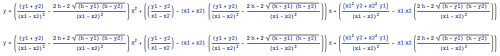However, no solution is complete without verifying the results. Therefore, we will check the vertex to make sure we are producing the correct results.

Verifying the Result

To calculate the coordinates for the vertex, we have to take the derivative of our functions and set them equal to zero and solve for $x$:

$x_{m1}=\frac{(x_1\,y_2+x_2\,y_2)-(x_1+x_2)\,\left(h-\sqrt{(h-y_1)(h-y_2)}\right)}{(y_1+y_2)-2\left(h-\sqrt{(h-y_1)(h-y_2)}\right)}$

$x_{m2}=\frac{(x_1\,y_2+x_2\,y_2)-(x_1+x_2)\,\left(h+\sqrt{(h-y_1)(h-y_2)}\right)}{(y_1+y_2)-2\left(h+\sqrt{(h-y_1)(h-y_2)}\right)}$

Now that we have the $x$ coordinates for the vertices, we plug them back into the equations for the parabolas and we get (verified with Mathematica):

$f_1\left(x_{1}\right) = y_1$
$f_1\left(x_{2}\right) = y_2$

$f_1\left(x_{m1}\right) = h$

and for the second parabola (verified with Mathematica):

$f_2\left(x_{1}\right) = y_1$
$f_2\left(x_{2}\right) = y_2$

$f_2\left(x_{m2}\right) = h$

Thus, we have proved / verified that the equations do indeed satisfy the conditions of the challenge as can be seen in the following graph:I have attached the Mathematica 7 file for this challenge to this post for those who want to see how I worked the problem. Enjoy!!!

EighthChallenge.zip

Edited by Daedalus

##### Share on other sites

Fantastic, sorry I'm new didn't know how to operate latex on this site. :|

Also, great catch on the  I had the 0= blah blah.... just missed it when I typed it into the text.

Thanks for the puzzle and the kindness.##### Share on other sites

Fantastic, sorry I'm new didn't know how to operate latex on this site. :|

Also, great catch on the  I had the 0= blah blah.... just missed it when I typed it into the text.

Thanks for the puzzle and the kindness.You're welcomeTo see how we use LaTeX, you can click on a formula and a pop-up will appear showing you the LaTeX. Basically, you enclose your equations with [ math ] [ \math ] tags (just remove the spaces in the tags. I had to add the space so that the forum will display the tags). You can also use the sandbox to test LaTeX expressions, and we have a tutorial on using LaTeX too.

Good job on solving the challenge!!!##### Share on other sites

Congrats mutatis! Like many say, you just won the internetAnd I feel like I owe an intellectual apology to Daedelus. I unreasonably assumed this would be like most math puzzles one is familiar with, short and competitive (quite wrong on that). It was pretty frustrating, but it felt really good to dive into a problem that actually takes up your time and forces you to think.

##### Share on other sites

Congrats mutatis! Like many say, you just won the internetAnd I feel like I owe an intellectual apology to Daedelus. I unreasonably assumed this would be like most math puzzles one is familiar with, short and competitive (quite wrong on that). It was pretty frustrating, but it felt really good to dive into a problem that actually takes up your time and forces you to think.

You're welcome Amaton. I try really hard to make sure I come up with interesting challenges that invoke thought and are challenging. A lot of my challenges are actual problems that I have thought of and solved myself at one point in time or another. That is why you can't find them on the internet or in math books.

Edited by Daedalus

##### Share on other sites

Amaton thanks for the props!

The ONLY thing I was able to find that remotely resembles the challenge was this: http://jliszka.github.io/2013/09/24/more-backwards-functions-unevaluating-polynomials.html

And it was only posted a few days after the challenge was made live...and it only resembles the problem in the sense that a star is similar to an LED...sure they both make light, but they're operating via completely different processes.

##### Share on other sites

What would make this challenge interesting is for each parabola found for one given parabola, you would also find the two parabolas for the found parabolas. If this were to be done, I would like to see how it looks.

##### Share on other sites

What would make this challenge interesting is for each parabola found for one given parabola, you would also find the two parabolas for the found parabolas. If this were to be done, I would like to see how it looks.

I'm not quite following what you are saying. Could you elaborate a little?

Edited by Daedalus

##### Share on other sites

I'm not quite following what you are saying. Could you elaborate a little?

Well, let's take the original image:The challenge was to find the two parabolas that are the height of the original parabola and that meet at both points. Now, once you find those two parabolas, take those two parabolas, specify the points on those parabolas with relatively the same position on those parabolas, and then find the two parabolas that go through those points.

## Create an account

Register a new account